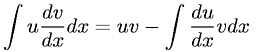Equations > Calculus > Integration/Integrals > Integration by parts

### Integration by partsLatex Code:

MathML Code:

 $\int u\frac\mathrm{dv}\mathrm{dx}\mathrm{dx}=\mathrm{uv}-\int \frac\mathrm{du}\mathrm{dx}\mathrm{vdx}$

MathType 5.0: